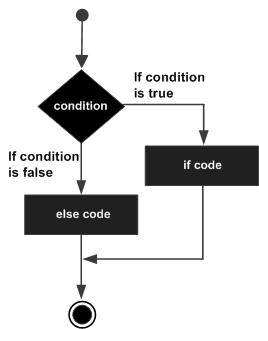# Swift - If... Else Statement

An if statement can be followed by an optional else statement, which executes when the Boolean expression is false.

## Syntax

The syntax of an if...else statement in Swift 4 is as follows −

```if boolean_expression {
/* statement(s) will execute if the boolean expression is true */

} else {
/* statement(s) will execute if the boolean expression is false */
}
```

If the Boolean expression evaluates to true, then the if block of code will be executed, otherwise else block of code will be executed.## Example

```var varA:Int = 100;

/* Check the boolean condition using if statement */
if varA < 20 {
/* If condition is true then print the following */
print("varA is less than 20");

} else {
/* If condition is false then print the following */
print("varA is not less than 20");
}

print("Value of variable varA is \(varA)");
```

When the above code is compiled and executed, it produces the following result −

```varA is not less than 20
Value of variable varA is 100
```
swift_decision_making.htm